## Length Mass Volume: Real Life 2-Step Word Problems

Practice Unlimited Questions

#### 1. Helen had some raisins. After packing them into 7 packets of 130 g each, she had 677 g of raisins left. Find the mass of raisins she had in the beginning. Give your answer in kilograms and grams.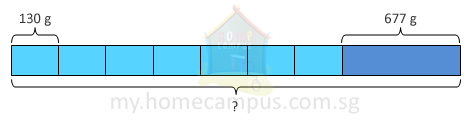Step 1:
130 g  ×  7  =  910 g
She packed 910 g of raisins altogether.

Step 2:
910 g  +  677 g
=  1587 g
=  1 kg 587 g
She had 1 kg 587 g of raisins in the beginning.

#### 2. Aadil is competing in a hurdle race. He has to run over hurdles from Point A to Point B and back. The distance between Point A and Point B is 5 km. How much further must he run to finish the race, if he has already run 6 km?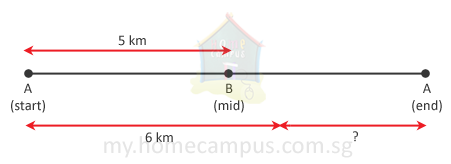Step 1:
5 km   ×   2   =   10 km
The total distance from Point A to Point B and back is 10 km.

Step 2:
10 km   −   6 km   =   4 km
He must run 4 km further to finish the race.

#### 3. Mr. Rogers had 13 empty pails, each of capacity 7 l. He also had a barrel containing 42 l of water. He poured out all the water from the barrel to completely fill some of the pails. In the end, how many pails were left empty?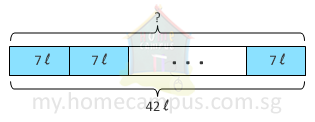Step 1:
42   ÷   7   =   6
42 l of water could fill 6 pails completely.

Step 2:
13   −   6   =   7
In the end, 7 pails were left empty.

#### 4. Sylvia has 5 balls of yarn. Each ball has 1 m 65 cm of yarn. She uses three balls of yarn for a craft project. How many centimetres of yarn has she left?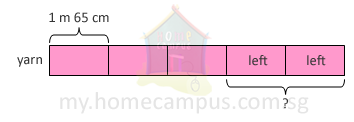Step 1:
5   −   3   =   2
She has 2 balls of yarn left.

Step 2:
1 m 65 cm   ×   2
=  165 cm   ×   2
=  330 cm
She has 330 cm of yarn left.

#### 5. A table has a mass of 16 kg. It is 8 times as heavy as a stool. Find the total mass of the table and the stool.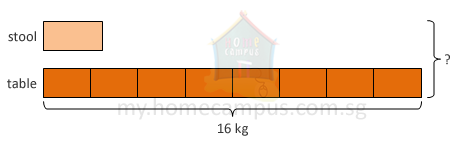Step 1:
16 kg   ÷   8   =   2 kg
The mass of the stool is 2 kg.

Step 2:
16 kg   +   2 kg   =   18 kg
The total mass of the table and the stool is 18 kg.

#### 6. Rashad has a flask containing 1070 ml of ink. He also has 3 bottles, each containing 75 ml of ink. He pours all the ink from the 3 bottles into the flask and fills it to the brim. Find the capacity of the flask in litres and millilitres.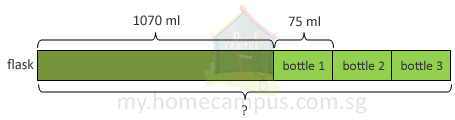Step 1:
75 ml   ×   3   =   225 ml
The 3 bottles contain a total of 225 ml of ink.

Step 2:
1070 ml   +   225 ml
=  1295 ml
=  1 l 295 ml
The capacity of the flask is 1 l 295 ml.